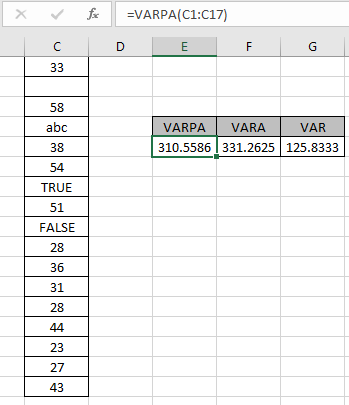# How to use the VARPA function in ExcelIn this article, we will learn how to use VARPA Function in Excel. VARPA function is a statistical function which returns the numerical variance value for the array of data given in as input. In simple words, get the estimated variation of population of the data. The VARPA function considers text values as 0, Logic values TRUE and FALSE as 1 and 0 respectively. Use VAR or newer VARP function to ignore all other values except numbers.

Syntax:

=VARPA(number1,number2,..)

number : number or array given as cell reference
Different VAR function in Excel and its usage.

 Name Data set Text and logicals VAR Sample Ignored VARP Population Ignored VAR.S Sample Ignored VAR.P Population Ignored VARA Sample Evaluated VARPA Population Evaluated

Example:
All of these might be confusing to understand. So, let's test this formula via running it on the example shown below. Here we have some numbers to test the VARPA function in Excel.
Use the formula:

=VARPA(C1:C17)

Here the array of values is given as cell reference. You can also input values as numbers.Here the function doesn't ignore blank cells, TRUE and FALSE logic values and text values. The VARPA function returns 310.5586 as population variance. The VARA function returns 331.2625 as sample variance considering text values as 0, TRUE value as 1 and FALSE value as 0 whereas VAR function returns 125.8333 as sample data ignoring all other variable types except numbers.

What is the Difference in VAR, VARS, VAR.S (all are same) and VARPA or VAR.P.
The denominatore in VAR, VARS,VAR.S is n-1. Where n is the number of observations. Where  VAR.P has denominator n.

 VAR/VARS/VAR.S VARP/VAR.P =summation[(x-x)2/(n-1)] =summation[(x-x)2/n]

VAR.S is used for population data. VAR.P is used for population data.

Here are some observational notes shown below.
Notes:

1. The formula only works with numbers, text and Logic values as argument.
2. Use the VAR, VARS and VAR.S function for the population data.
3. The VARPA function considers text, logic values and blank cells.

Hope this article about How to use the VARPA function in Excel is explanatory. Find more articles on statistical function formulas here. If you liked our blogs, share it with your fristarts on Facebook. And also you can follow us on Twitter and Facebook. We would love to hear from you, do let us know how we can improve, complement or innovate our work and make it better for you. Write to us at info@exceltip.com.

Related Articles

How to use the VAR function in Excel : Calculate the variance for the sample data numbers in excel using the VAR function

How to use the VAR.P function in Excel : Calculate the variance for the population data numbers in excel using the VAR.P function

How to use the DVAR function in Excel : Calculate the variance for the sample data numbers having multiple criteria in excel using the DVAR function

How to Use STDEV Function in Excel : Calculate the standard deviation for the data numbers in excel using the STDEV function

Regressions Analysis in Excel : Regression is an Analysis Tool, which we use for analyzing large amounts of data and making forecasts and predictions in Microsoft Excel.

How to Create Standard Deviation Graph : The standard deviation tells how much the data is clustered around the mean of the data.

Popular Articles:

50 Excel Shortcuts to Increase Your Productivity | Get faster at your task. These 50 shortcuts will make you work even faster on Excel.

The VLOOKUP Function in Excel | This is one of the most used and popular functions of excel that is used to lookup values from different ranges and sheets.

COUNTIF in Excel 2016 | Count values with conditions using this amazing function. You don't need to filter your data to count specific values. Countif function is essential to prepare your dashboard.

How to Use SUMIF Function in Excel | This is another dashboard essential function. This helps you sum up values on specific conditions.

Terms and Conditions of use

The applications/code on this site are distributed as is and without warranties or liability. In no event shall the owner of the copyrights, or the authors of the applications/code be liable for any loss of profit, any problems or any damage resulting from the use or evaluation of the applications/code.